# score_transformation¶

## Module: missions.nodes.postprocessing.score_transformation¶

Transform the classification score (especially the one of the SVM)

Inheritance diagram for pySPACE.missions.nodes.postprocessing.score_transformation: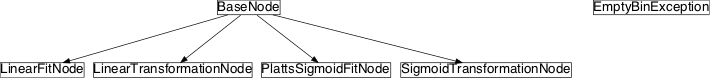## Class Summary¶

 EmptyBinException PlattsSigmoidFitNode([priors, class_labels, ...]) Map prediction scores to probability estimates with a sigmoid fit SigmoidTransformationNode([class_labels, A, ...]) Transform score to interval [0,1] with a sigmoid function LinearTransformationNode([class_labels, ...]) Scaling and offset shift, and relabeling due to new decision boundary LinearFitNode([class_labels]) Linear mapping between score and [0,1]

## Classes¶

### EmptyBinException¶

class pySPACE.missions.nodes.postprocessing.score_transformation.EmptyBinException[source]

Bases: exceptions.Exception

__weakref__

list of weak references to the object (if defined)

### PlattsSigmoidFitNode¶

class pySPACE.missions.nodes.postprocessing.score_transformation.PlattsSigmoidFitNode(priors=None, class_labels=[], oversampling=False, store_plots=False, store_probabilities=False, **kwargs)[source]

Map prediction scores to probability estimates with a sigmoid fit

This node uses a sigmoid fit to map a prediction score to a class probability estimate, i.e. a value between 0 and 1, where e.g. 0.5 means 50% probability of the positive class which must not necessarily correspond to a SVM score of 0. For more information see ‘Probabilistic Outputs for Support Vector Machines and Comparisons to Regularized Likelihood Methods’ (Platt) 1999. The parametric form of the sigmoid function is: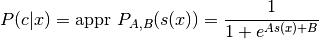where c is the actual class, x the data, s(x) the prediction score and A and B are calculated through the training examples.

Note

Learning this transformation on the same training data than the classifier was trained is not recommended for non-linear kernels (due to over-fitting).

The best parameter setting z*=(A*,B*) is determined by solving the following regularized maximum likelihood problem: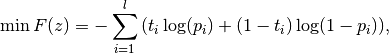for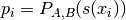and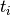are target probabilities defined according to priors and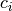.

The implementation is improved to ensure convergence and to avoid numerical difficulties (see ‘A Note on Platt’s Probabilistic Outputs for Support Vector Machines’ (HT Lin, RC Weng) 2007).

Parameters

priors: class_labels: A tuple that consists the number of examples expected for each class (first element negative class, second element positive class). If the parameter is not specified, the numbers in the training set are used. (optional, default: None) Determines the order of classes, i.e. the mapping of class labels onto integers. The first element of the list should be the negative class, the second should be the positive class. If this parameter is not specified, the order is determined based on the order of occurrence in the training data (which is more or less arbitrary). (optional, default: []) If True different class distributions are balanced by oversampling and random drawing where appropriate (if the overrepresented class is not divisible by the underrepresented class). (optional, default: False) If True ‘reliable diagrams’ of the training and test data are stored. A discretization of the scores is made to calculate empirical probabilities. The number of scores per bin is displayed on every data point in the figure and shows how accurate the estimate is (the higher the number the better). If the fit is reliable the empirical probabilities should scatter around the diagonal in the right plots. Although the store variable is set to True if this variable is set. If True the calculated probability and the corresponding label for each prediction is pickeled and saved in the results directory. Although the store variable is set to True if this variable is set. (optional, default: False) If True store_plots and store_probabilities are set to True. This is the “simple” way to store both the plots and the probabilities.

Exemplary Call

-
node : PSF
parameters :
class_labels : ['Target','Standard']

POSSIBLE NODE NAMES:

• PlattsSigmoidFitNode
• PlattsSigmoidFit
• PSF
POSSIBLE INPUT TYPES:

• PredictionVector

Class Components Summary

 _discretize(predictions, labels[, bins]) Discretize predictions into bins. _empirical_probability(l_discrete) Return dictionary of empirical class probabilities for discretized label list. _execute(x) Evaluate each prediction with the sigmoid mapping learned. _stop_training() Compute parameter A and B for sigmoid fit. _train(data, class_label) Collect SVM output and true labels. input_types is_supervised() is_trainable() store_state(result_dir[, index]) Stores plots of score distribution and sigmoid fit or/and the calculated probabilities with the corresponding label.
__init__(priors=None, class_labels=[], oversampling=False, store_plots=False, store_probabilities=False, **kwargs)[source]
is_trainable()[source]
is_supervised()[source]
_train(data, class_label)[source]

Collect SVM output and true labels.

_stop_training()[source]

Compute parameter A and B for sigmoid fit.

_execute(x)[source]

Evaluate each prediction with the sigmoid mapping learned.

_discretize(predictions, labels, bins=12)[source]

Discretize predictions into bins.

Return bin scores and 2d list of discretized labels.

_empirical_probability(l_discrete)[source]

Return dictionary of empirical class probabilities for discretized label list.

store_state(result_dir, index=None)[source]

Stores plots of score distribution and sigmoid fit or/and the calculated probabilities with the corresponding label.

input_types = ['PredictionVector']

### SigmoidTransformationNode¶

class pySPACE.missions.nodes.postprocessing.score_transformation.SigmoidTransformationNode(class_labels=['Standard', 'Target'], A=-1, B=0, offset=None, **kwargs)[source]

Transform score to interval [0,1] with a sigmoid function

The new decision border will be at 0.5.

Warning

This is NOT a probability mapping and parameters should be set for the function.

This node is intended to be externally optimized, such that it generalizes the threshold optimization for soft metrics.

The used sigmoid fit function is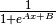. It is 0.5 at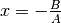.

Parameters

A: Scaling of prediction value. See above. (optional, default: -1) Shifting of scaled prediction. See above. (optional, default: 0) Has the meaning of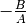and replaces the parameter B if used. (optional, default: None) Determines the order of classes, i.e. the mapping of class labels onto integers. The first element of the list should be the negative class, the second should be the positive class. In the context positive should be the class mapped greater than 0.5 and the other class should be the negative one. If the original prediction value had the same orientation, A should be chosen negative. (optional, default: [‘Standard’,’Target’])

Exemplary Call

-
node : SigTrans
parameters :
class_labels : ['Standard','Target']

POSSIBLE NODE NAMES:

• SigmoidTransformationNode
• SigmoidTransformation
• SigTrans
POSSIBLE INPUT TYPES:

• PredictionVector

Class Components Summary

 _execute(data) Evaluate each prediction with the sigmoid mapping learned. input_types is_supervised() is_trainable()
input_types = ['PredictionVector']
__init__(class_labels=['Standard', 'Target'], A=-1, B=0, offset=None, **kwargs)[source]
is_trainable()[source]
is_supervised()[source]
_execute(data)[source]

Evaluate each prediction with the sigmoid mapping learned.

### LinearTransformationNode¶

class pySPACE.missions.nodes.postprocessing.score_transformation.LinearTransformationNode(class_labels=None, offset=0, scaling=1, decision_boundary=None, **kwargs)[source]

Scaling and offset shift, and relabeling due to new decision boundary

Having a prediction value x it is mapped to (x+*offset*)*scaling*. If the result is lower than the decision boundary it is mapped to the first class label for the negative class and otherwise to the second positive class.

Parameters

class labels: offset: This mandatory parameter defines the ordering of class labels for the mapping after the transformation. If this parameter is not specified, the label remains unchanged. This is for example feasible for regression mappings. Note This parameter could be also used to change class label strings, but this would probably cause problems in the evaluation step. (recommended, default: None) Shift of the prediction value. (optional, default: 0) Scaling factor applied after offset shift. (optional, default: 1) Everything lower this value is classified as class one and everything else as class two. By default no labels are changed.

Exemplary Call

-   node : LinearTransformation
parameters :
class_labels : ['Standard', 'Target']
offset : 1
scaling : 42
decision_boundary : 3

POSSIBLE NODE NAMES:

• LinearTransformation
• LinearTransformationNode
POSSIBLE INPUT TYPES:

• PredictionVector

Class Components Summary

 _execute(x) (x+o)*s < d input_types
__init__(class_labels=None, offset=0, scaling=1, decision_boundary=None, **kwargs)[source]
_execute(x)[source]

(x+o)*s < d

input_types = ['PredictionVector']

### LinearFitNode¶

class pySPACE.missions.nodes.postprocessing.score_transformation.LinearFitNode(class_labels=[], **kwargs)[source]

Linear mapping between score and [0,1]

This node maps the unbounded SVM score linear to bound it between [0,1]. If the result can be interpreted as probability can be seen in the reliable diagrams.

Parameters

class_labels: Determines the order of classes, i.e. the mapping of class labels onto integers. The first element of the list should be the negative class, the second should be the positive class. If this parameter is not specified, the order is determined based on the order of occurrence in the training data (which is more or less arbitrary). (optional, default: []) If True ‘reliable diagrams’ of the training and test data are stored. A discretization of the scores is made to calculate empirical probabilities. The number of scores per bin is displayed on every data point in the figure and shows how accurate the estimate is (the higher the number the better). If the fit is reliable the empirical probabilities should scatter around the diagonal in the right plots.

Exemplary Call

-
node : LinearFit
parameters :
class_labels : ['Standard','Target']

POSSIBLE NODE NAMES:

• LinearFitNode
• LinearFit
POSSIBLE INPUT TYPES:

• PredictionVector

Class Components Summary

 _discretize(predictions, labels[, bins]) Discretize predictions into bins. _empirical_probability(l_discrete) Return dictionary of empirical class probabilities for discretized label list. _execute(x) Evaluate each prediction with the linear mapping learned. _stop_training() Compute max range of the score according to the class. _train(data, class_label) Collect SVM output and true labels. input_types is_supervised() is_trainable() store_state(result_dir[, index]) Stores plots of score distribution and sigmoid fit.
__init__(class_labels=[], **kwargs)[source]
is_trainable()[source]
is_supervised()[source]
_train(data, class_label)[source]

Collect SVM output and true labels.

_stop_training()[source]

Compute max range of the score according to the class.

_execute(x)[source]

Evaluate each prediction with the linear mapping learned.

_discretize(predictions, labels, bins=12)[source]

Discretize predictions into bins. Return bin scores and 2d list of discretized labels.

_empirical_probability(l_discrete)[source]

Return dictionary of empirical class probabilities for discretized label list.

store_state(result_dir, index=None)[source]

Stores plots of score distribution and sigmoid fit.

input_types = ['PredictionVector']